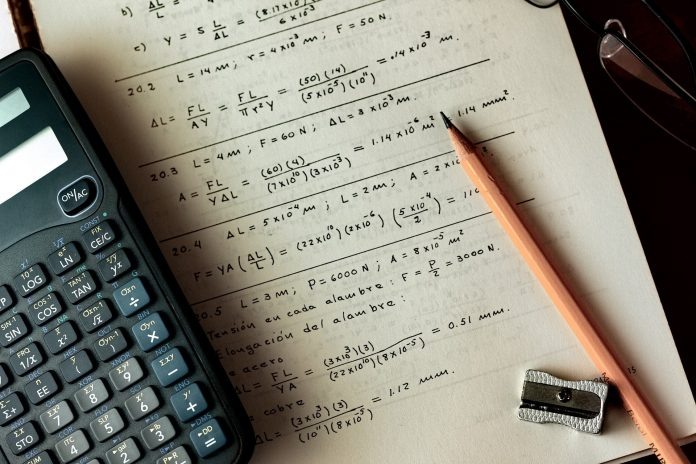# PEMDAS Rule – History & Introduction to Order of Operations

0We do operations such as addition, subtraction, multiplication, and division in mathematics. These activities are carried out according to a set of rules, or to put it another way, there is an order of operations. The PEMDAS rule is one of the rules that is identical to the BODMAS rule. PEMDAS is written in its entire form as follows:

The letter P stands for Parentheses which denote the different types of brackets used in mathematics [{()}], the letter E stands for Exponents (Powers as well as Roots), the letters MD stand for Multiplication and Division (Order to be kept in mind – left to right) (× and ÷), the letters AS stand for Addition and Subtraction (left to right) (+ and -).

In this article, we are going to discuss the PEMDAS Rule in detail as well as how the order of operations is used in maths while we solve equations.

## What is the Order of Operations in Mathematics?

The sequence or the order of operations informs us how to solve steps in expressions with several operations in the correct order. We begin by resolving any operations included within parenthesis or brackets. Second, any exponents are solved. Third, we work our way through all multiplication and division problems from left to right. Fourth, we work from left to right to answer all addition and subtraction problems.

## Importance of Order of Operations

The order of operations is a rule that shows you how to measure distinct elements of a math problem in the correct order. (Operation is originally known as “calculation.”) Operations include things like subtraction, multiplication, and division.)

The order of operations is important. It ensures that everyone can read and solve a problem in a common way. Formulas for real-world calculations in finance and science would be useless without a consistent sequence of operations, and it would be impossible to determine if you were obtaining the right answer on a math test!

## What is the PEMDAS Rule?

The letters PEMDAS stand for parenthesis, exponents, multiplication, division, addition, and subtraction. The order of the letters in PEMDAS informs you what to calculate first, second, third, and so on, until the computation is complete, given two or more operations in a single statement. If the equation contains grouping symbols, PEMDAS instructs you to compute within the grouping symbols first.

Because the letters PEMDAS and the phrases parenthesis, exponents, multiplication, division, addition, and subtraction may be difficult to recall, a sentence has been added to the letters in PEMDAS: Please Excuse My Dear Aunt Sally. It may be simpler to recall the PEMDAS sequence of operations if you can remember this phrase.

## Examples

1. 21/ 7 *12/2 is an equation that you have to solve.

Calculate from the left to the right side because ‘Multiplication’ and ‘Division’ perform equally well. Solve 21/ 7 equals 3, then 3 multiplied by 12 equals 36, and finally 36 divided 2 equals 18.

Calculate ‘Addition’ and ‘Subtraction’ at the end (half) of the equation. When addition and subtraction occur in the same portion of the equation, their order in the given part of the equation is calculated from left to right.

1. 5+10-2+5

Since we have already discussed that subtraction, as well as addition, are performed equally, we need to solve the given equation from left to right. Let’s begin!

We will first solve 5+10 = 15 , then we will solve 15-2 = 13 and then we will solve 13+5 = 18.

If you want to go through a few more examples and if you want to understand the topic in a much more interesting manner you can visit the Cuemath website for the same.